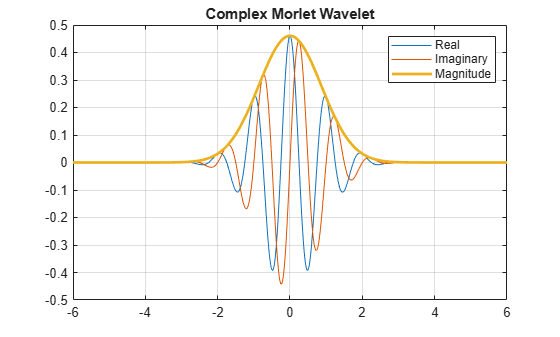Main Content

# cmorwavf

Complex Morlet wavelet

## Syntax

``[psi,x] = cmorwavf(lb,ub,n)``
``[psi,x] = cmorwavf(lb,ub,n,fb,fc)``

## Description

````[psi,x] = cmorwavf(lb,ub,n)` returns the complex Morlet wavelet, `psi`, with time-decay parameter, `fb`, and center frequency, `fc`, both equal to 1. The wavelet is evaluated on an `n`-point regular grid, `x`, for the interval [`lb`,`ub`]. The general expression for the complex Morlet wavelet is $\psi \left(x\right)=\frac{1}{\sqrt{\pi \cdot \text{fb}}}\mathrm{exp}\left(2\pi i\cdot \text{fc}\cdot x\right)\mathrm{exp}\left(-{x}^{2}/\text{fb}\right).$```

example

````[psi,x] = cmorwavf(lb,ub,n,fb,fc)` returns the complex Morlet wavelet with time-decay parameter, `fb`, and center frequency, `fc`.```

## Examples

collapse all

Construct a complex-valued Morlet wavelet with a bandwidth parameter of 1.5 and a center frequency of 1. Set the effective support to $\left[-8,8\right]$ and the length of the wavelet to 1000.

```N = 1000; Lb = -8; Ub = 8; fb = 1.5; fc = 1; [psi,x] = cmorwavf(Lb,Ub,N,fb,fc);```

Plot the real and imaginary parts of the wavelet.

```subplot(2,1,1) plot(x,real(psi)); title('Real Part'); subplot(2,1,2) plot(x,imag(psi)); title('Imaginary Part');```This example shows how the complex Morlet wavelet shape in the frequency domain is affected by the value of the bandwidth parameter (`Fb`). Both wavelets have a center frequency of 1. One wavelet has an `Fb` value of 0.5 and the other wavelet has a value of 8.

```f = -5:.01:5; Fc = 1; Fb1 = 0.5; Fb2 = 8; psihat1 = exp(-pi^2*Fb1*(f-Fc).^2); psihat2 = exp(-pi^2*Fb2*(f-Fc).^2); plot(f,psihat1) hold on; plot(f,psihat2,'r') legend('Fb = 0.5','Fb = 8')```The `Fb` bandwidth parameter for the complex Morlet wavelet is the inverse of the variance in frequency. Therefore, increasing Fb results in a narrower concentration of energy around the center frequency.

## Input Arguments

collapse all

Left endpoint of the closed interval, specified as a scalar. `lb` is strictly less than `ub`.

Right endpoint of the closed interval, specified as a scalar. `ub` is strictly greater than `ub`.

Number of regularly spaced points in the interval [`lb`,`ub`], specified as a positive integer.

Time-decay parameter, specified as a positive scalar. `fb` controls the decay in the time domain and the corresponding energy spread (bandwidth) in the frequency domain. `fb` is the inverse of the variance in the frequency domain.

• Increasing `fb` makes the wavelet energy more concentrated around the center frequency and results in slower decay of the wavelet in the time domain.

• Decreasing `fb` results in faster decay of the wavelet in the time domain and less energy spread in the frequency domain.

The value of `fb` does not affect the center frequency. When converting from scale to frequency, only the center frequency affects the frequency values. The energy spread or bandwidth parameter affects how localized the wavelet is in the frequency domain.

Center frequency, specified as a positive scalar.

## Output Arguments

collapse all

Complex Morlet wavelet, returned as a complex-valued 1-by-`n` vector.

Sample points where the complex Morlet vector is evaluated, returned as a 1-by-`n` vector. The sample points are evenly distributed between `lb` and `ub`.

 Teolis, Anthony. Computational Signal Processing with Wavelets. Boston, MA: Birkhäuser Boston, 1998. https://doi.org/10.1007/978-1-4612-4142-3.

## Support#### Try MATLAB, Simulink, and Other Products

Get trial now